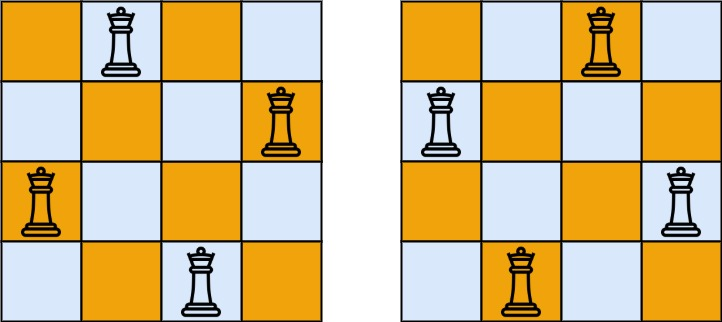# 51. N-Queens | LeetCode Solution

## 51. N-Queens | LeetCode Solution

The n-queens puzzle is the problem of placing `n` queens on an `n x n` chessboard such that no two queens attack each other.

Given an integer `n`, return all distinct solutions to the n-queens puzzle. You may return the answer in any order.

Each solution contains a distinct board configuration of the n-queens' placement, where `'Q'` and `'.'` both indicate a queen and an empty space, respectively.

Example 1:```Input: n = 4
Output: [[".Q..","...Q","Q...","..Q."],["..Q.","Q...","...Q",".Q.."]]
Explanation: There exist two distinct solutions to the 4-queens puzzle as shown above
```

Example 2:

```Input: n = 1
Output: [["Q"]]
```

Constraints:

• `1 <= n <= 9`

class Solution {
public:
vector<vector<string>>res;

bool check(int r,int c,vector<string>&v,int n){
for(int i=0; i<r; i++){
if(v[i][c]=='Q')return false;
}
int k=r;
for(int i=r-1,j=c-1; i>=0 && j>=0 ; i--,j--){
if(v[i][j]=='Q')return false;
}
for(int i=r-1,j=c+1; i>=0 && j<n ; i--,j++){
if(v[i][j]=='Q')return false;
}

return true;
}

void helper(int n, int row,int col,vector<string>&v){
if(row>=n){
res.push_back(v);
return;
}

for(int i=col; i<n; i++){
if(check(row,i,v,n)){v[row][i]='Q';
helper(n,row+1,0,v);
v[row][i]='.';
}
}
}

vector<vector<string>> solveNQueens(int n) {
vector<string>ans(n,string(n,'.'));
// res.push_back(ans);
helper(n,0,0,ans);
return res;
}
};